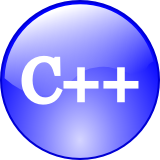## C/C++ Implementation for Longest Common Substring AlgorithmThe longest common substring problem is to find the longest string that is a substring of two or more given strings.

You can build a generalized suffix tree for a set of strings with multiple strings using this implementation. A suffix tree contains all the suffixes of the given text as their keys and positions in the text as their values.

```string longestCommonSubstring(const string& str1, const string& str2)
{

if(str1.empty() || str2.empty())
{
return 0;
}

int *curr = new int [str2.size()];
int *prev = new int [str2.size()];
int *swap = NULL;
int maxSubstr = 0;
string longest;

for(unsigned int i = 0; i < str1.size(); ++i)
{
for(unsigned int j = 0; j < str2.size(); ++j)
{
if(str1[i] != str2[j])
{
curr[j] = 0;
}
else
{
if(i == 0 || j == 0)
{
curr[j] = 1;
}
else
{
curr[j] = 1 + prev[j-1];
}

if(maxSubstr < curr[j])
{
maxSubstr = curr[j];
longest.clear();
}

if (maxSubstr == curr[j])
{
longest += str1.substr(i - maxSubstr + 1, i + 1);
}
}
}
swap=curr;
curr=prev;
prev=swap;
}
delete [] curr;
delete [] prev;
return longest.substr(0, maxSubstr);
}

```

## C/C++ Implementation of Levenshtein Distance Algorithm for Approximate String MatchingThe Levenshtein is a measure of how costly it is to adapt a string into another one. If you assign a cost to adding a single character, switching one character for another, and removing a character then you can compute the cost between any two given strings.

Changing a character can be seen as removing a char and adding another one so when adding has cost 1 and removing has cost of one a modification has cost of 2.

The difference between two strings can also be measured in terms of the Levenshtein distance: the distance measure if you think the cost as the “distance” between two strings.

Text comparison is becoming an ever more relevant matter for many fast growing areas such as information retrieval, computational biology, online searching. Levenshtein distance can be used mostly to edit distance, explaining the problem and its relevance.

```int levDistance(const std::string source, const std::string target)
{

// Step 1

const int n = source.length();
const int m = target.length();
if (n == 0) {
return m;
}
if (m == 0) {
return n;
}

// Good form to declare a TYPEDEF

typedef std::vector< std::vector > Tmatrix;

Tmatrix matrix(n+1);

// Size the vectors in the 2.nd dimension. Unfortunately C++ doesn't
// allow for allocation on declaration of 2.nd dimension of vec of vec

for (int i = 0; i <= n; i++) {
matrix[i].resize(m+1);
}

// Step 2

for (int i = 0; i <= n; i++) {
matrix[i]=i;
}

for (int j = 0; j <= m; j++) {
matrix[j]=j;
}

// Step 3

for (int i = 1; i <= n; i++) {

const char s_i = source[i-1];

// Step 4

for (int j = 1; j <= m; j++) {

const char t_j = target[j-1];

// Step 5

int cost;
if (s_i == t_j) {
cost = 0;
}
else {
cost = 1;
}

// Step 6

const int above = matrix[i-1][j];
const int left = matrix[i][j-1];
const int diag = matrix[i-1][j-1];
int cell = min( above + 1, min(left + 1, diag + cost));

// Step 6A: Cover transposition, in addition to deletion,
// insertion and substitution. This step is taken from:
// Berghel, Hal ; Roach, David : "An Extension of Ukkonen's
// Enhanced Dynamic Programming ASM Algorithm"
// (http://www.acm.org/~hlb/publications/asm/asm.html)

if (i>2 && j>2) {
int trans=matrix[i-2][j-2]+1;
if (source[i-2]!=t_j) trans++;
if (s_i!=target[j-2]) trans++;
if (cell>trans) cell=trans;
}

matrix[i][j]=cell;
}
}

// Step 7

return matrix[n][m];
}
```

## C/C++ Functions to Convert to UPPER CASE and lower caseC and C++ implementations that follow the standard library provide two functions in the header ctype.h to convert to upper and lower cases.

```char upperA = toupper('x');
char lowerA = tolower('X');
```

But if you want to write your own function to convert cases, here are two functions that use the string.h header.

## C and C++ Functions to Convert to lower case

```string lowercase(string s)
{
for (unsigned int i = 0; i < s.size(); i++)
if (s[i] >= 0x41 && s[i] <= 0x5A)
s[i] = s[i] + 0x20;
return s;
}
```

## C and C++ Functions to Convert to UPPER CASE

```string uppercase(string s)
{
for (unsigned int i = 0; i < s.size(); i++)
if (s[i] >= 0x61 && s[i] <= 0x7A)
s[i] = s[i] - 0x20;
return s;
}
```